# The framework of machine learning

Last updated on：2 years ago

I have learnt machining learning for around 3 years. However, I still misunderstood some basic theories of ML. Today, Dave wanted to assume ML.

# Basic concept

Machine learning is the field of study that gives computers the ability to learn without being explicitly programmed.

labelled data: you know the right answer to a question related to the data

unlabelled data: by contrast

classification: use predefined classes in which object is assigned

clustering: identify similarities between objects

The main advantage of using machine learning is that, once an algorithm learns what to do with data, it can do its work automatically.

Different sets in ML and DL

Data distribution sample:

Cases Train set Development set Test set
Small dataset ( with mistake) 70% 30%
small dataset 60% 20% 20%
large dataset 98% 1% 1%

Dev is used to choose algorithm, and used during evaluation of your classifier with different configurations or, variations in the feature representation, can be a bit biased. Test set is used to evaluate how well the DL is doing.

Not having a test set might be okay. Test set gives you a unbiased estimate of the performance of your final network (only dev set) . But make sure dev and test come from same distribution.

# Learning model

Different learning models are utilized to solve different types of problems.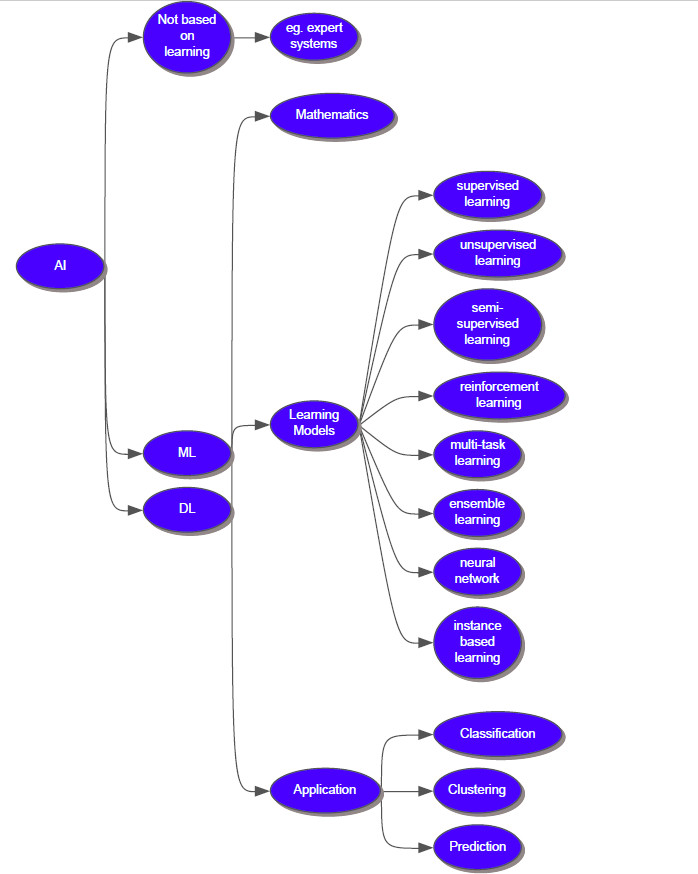## Supervised Learning

In supervised learning (SL), the machine is given a dataset (i.e., a set of data points), along with the right answers to a question corresponding to the data points. Given labelled data for each example in the data.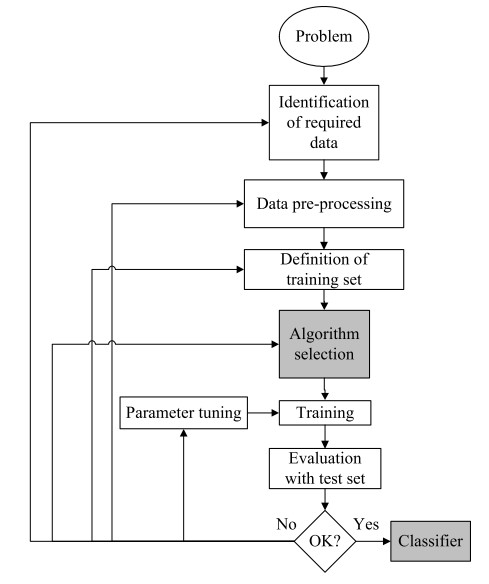### Problems

Classification problem: classify something into a distinct set of classes or categories

Regression problem: predict values of a continuous variable/ real-valued output

### Algorithms

Decision tree: sorting them based on their values.

Entropy is amount of information is needed to accurately describe the some sample.

$$Entropy(D) = - \sum_{i=1}^n p_i\times log(p_i)$$

where, D is a sample space.

$$Gain(D,a) = Entropy(D) - \sum_{i=1}^V \frac{|D^v |}{|D|}Entropy(D^v)$$

where, a is sparse property, with V possible values ${a^1, a^2, …, a^V}$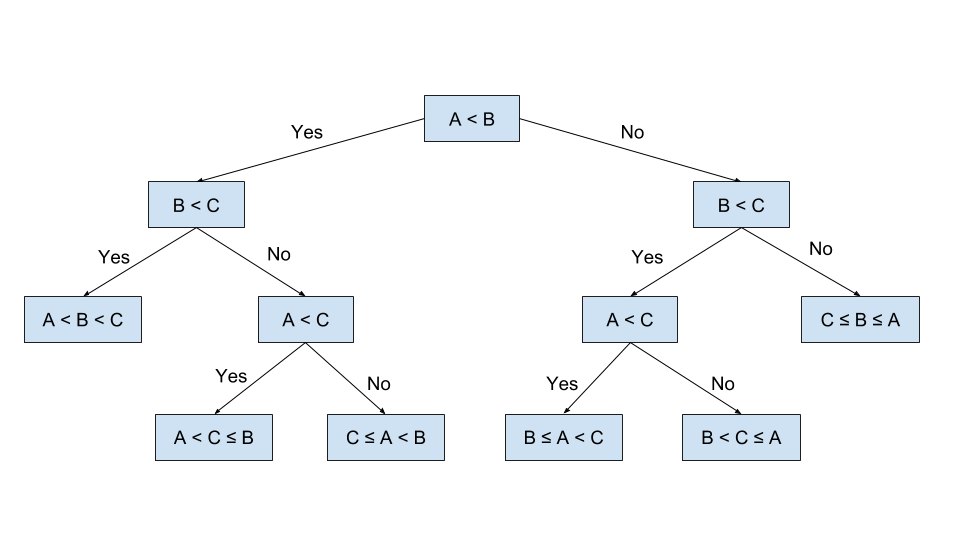Naive Bayes: It is mainly used for clustering and classification purpose.

$$P(c_i|A) = \frac{P(A|c_i)P(c_i)}{P(A)}$$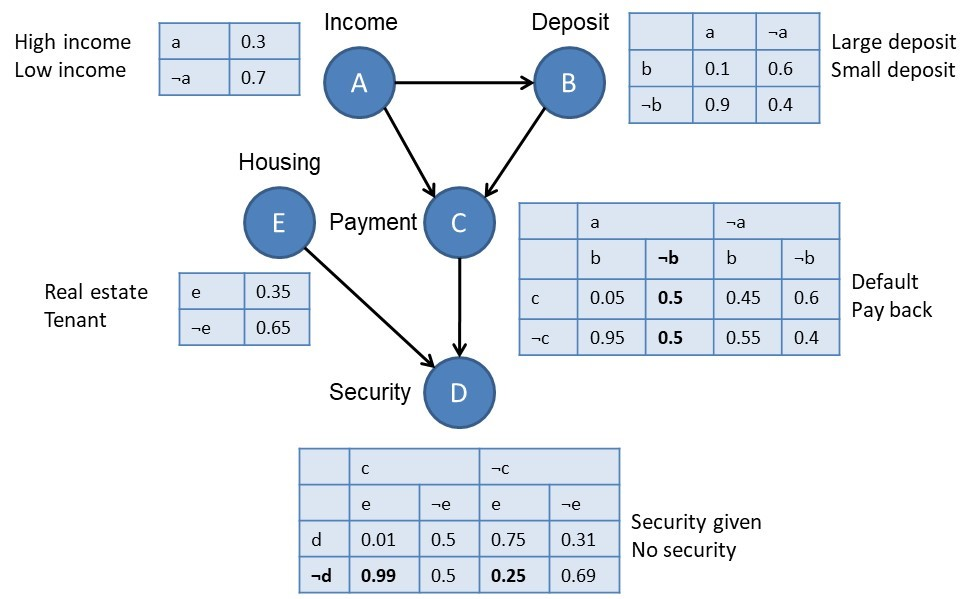I think Naive Bayes can be reformed into a decision tree.

Support vector machine: It is mainly used for classification.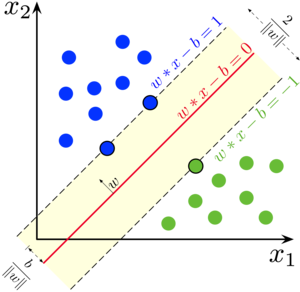## Unsupervised Learning

In unsupervised learning, the machine is provided with a set of data and is not provided with any right answer.
UL identify clusters or groups of similar items or similarity of the new item with an existing group, etc.

### Problems

Clustering: provides detail on the workings of unsupervised learning.

Feature reduction

### Algorithms

K-Mean clustering: Clustering or grouping is a type of unsupervised learning technique that when initiates, creates groups automatically

The items which possess similar characteristics are put in the same cluster.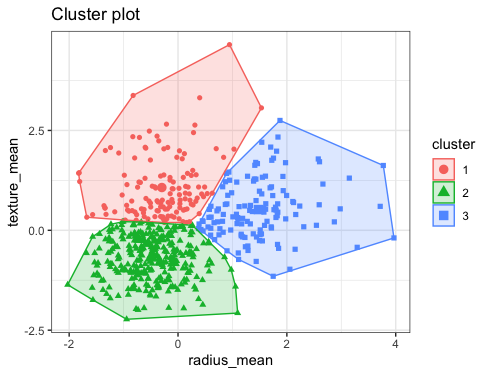Principle component analysis: The dimension of the data is reduced to make the computations faster and easier.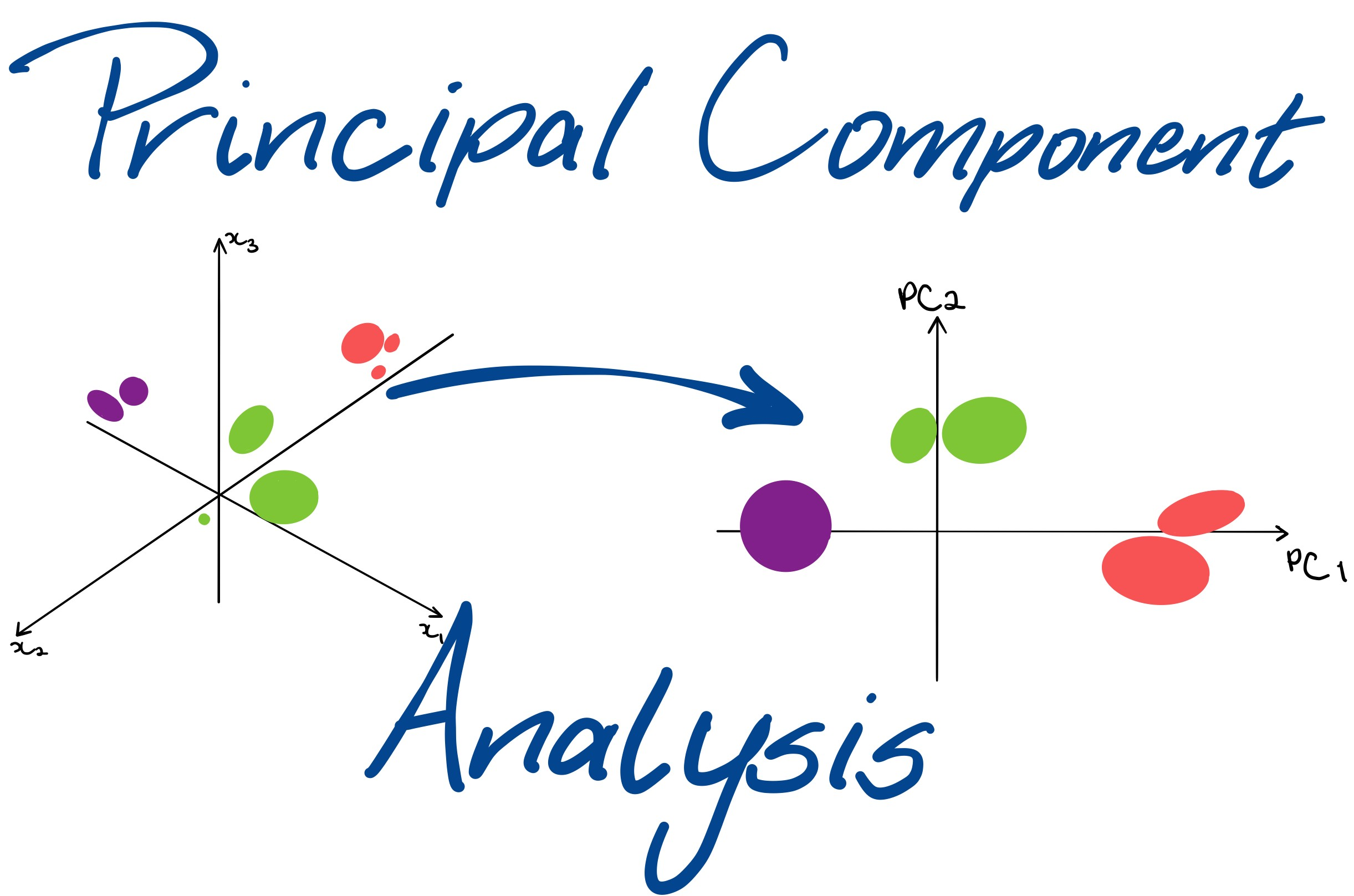## Semi-supervised Learning

Semi-supervised learning falls somewhere between supervised and unsupervised learning. Only a few data points are labelled.

You don’t have to spend a lot of time and effort in labelling each data point

### Algorithms

Generative models: assumes a structure like p(x,y) = p(y)p(x|y) where p(x|y) is a mixed distribution. e.g. Gaussian mixture models.

Within the unlabelled data, the mixed components can be identifiable.

Self-training: a classifier is trained with a portion of labelled data. The classifier is then fed with unlabelled data. The unlabelled points and the predicted labels are added together in the training set.

Transductive SVM: an extension of SVM, the labelled and unlabelled data both are considered. It is used to label the unlabelled data in such a way that the margin is maximum between the labelled and unlabelled data.

## Reinforcement Learning

Makes decisions based on which actions to take such that the outcome is more positive.

The agent receives input $i$, current state s, state transition r and input function I from the environment. Based on these inputs, the agent generates a behaviour B and takes an action a which generates an outcome.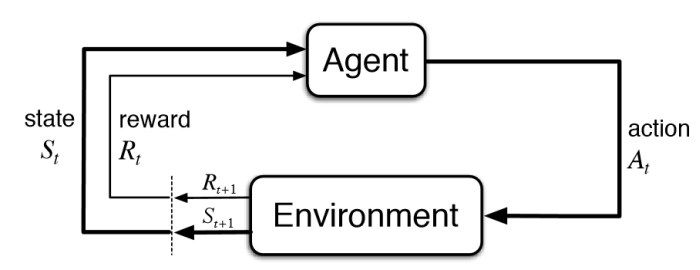### Problems

Changing situations: external situation (or, opponent’s play) is continuously changing, and the response from the machine has to consider the changing environment. eg. driving, the game of chess, backgammon.

Huge state space: Games like chess have almost infinite possible board configurations.

The simple goal of helping other learners to perform better.

## Ensemble learning

When various individual learners are combined to form only one learner then that particular type of learning is called ensemble learning.

### Problems

classification and regression

### Algorithms

Boosting: creates a collection of weak learners and convert them into one strong learner. Decrease bias and variance.

Bagging: is applied where the accuracy and stability of a machine learning algorithm need to be increased.

## Neural network learning

Artificial neural network or ANN is derived from the biological concept of neurons.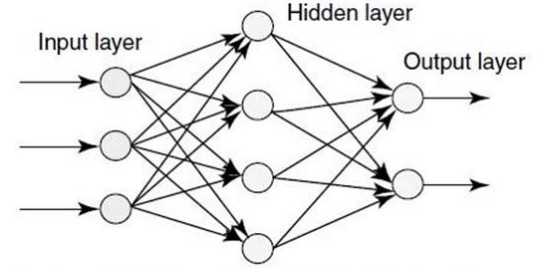### Algorithms

Supervised neural network: the output of the input is already known.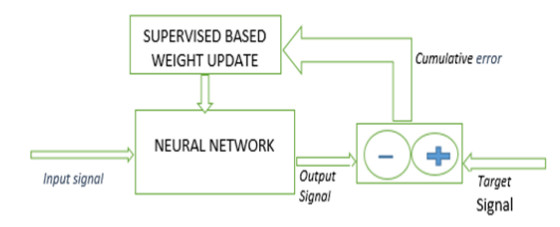Unsupervised neural network: has no prior clue about the output the input.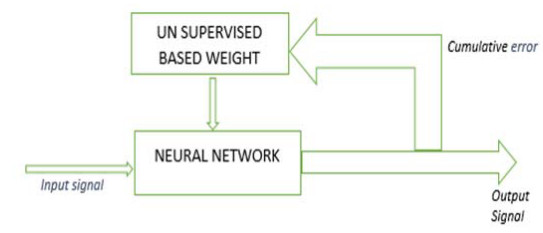Reinforcement neural network: the network behaves as if a human communicates with the environment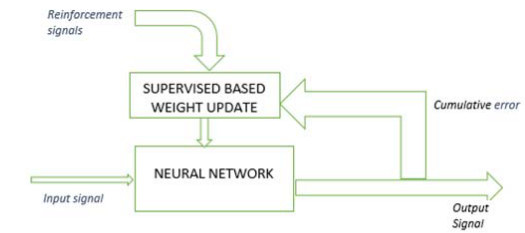# Reference

 Rebala, G., Ravi, A. and Churiwala, S., 2019. Machine Learning Definition and Basics. In An Introduction to Machine Learning. Springer, Cham.

 Dey, A., 2016. Machine learning algorithms: a review. International Journal of Computer Science and Information Technologies, 7(3), pp.1174-1179.

 Kotsiantis, S.B., Zaharakis, I. and Pintelas, P., 2007. Supervised machine learning: A review of classification techniques. Emerging artificial intelligence applications in computer engineering, 160(1), pp.3-24.

 Andrew Ng, Deep learning